# SAT Math Multiple Choice Question 827: Answer and Explanation

### Test Information

Question: 827

2.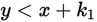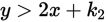Suppose that on a coordinate plane, (0, 0) is a solution to the system of inequalitiesgiven above. Which of the following conclusions about k1 and k2 must be true?

• A.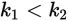• B.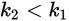• C.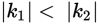• D.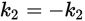Explanation:

B

Difficulty: Hard

Category: Heart of Algebra / Inequalities

Strategic Advice: Quickly examine the answer choices-there are no x's and no y's. This is a hint to plug in the solution (0, 0), which will eliminate both x and y.

Getting to the Answer: You are given that (0, 0) is a solution to the system of inequalities, which means that substituting 0 for x and 0 for y into each inequality must yield a true statement. You can then compare the values of k1 and k2.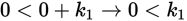, or k1 > 0, which means k1 is a positive number.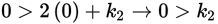, or k2 < 0, which means k2 is a negative number.

Every negative number is less than every positive number, which means k2 < k1, which is (B). (Note: Choice A is always false because it contradicts (B); C and D may or may not be true, depending on the values of k1 and k2, but the question asks which statement must be true.)